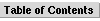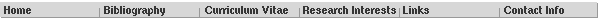Jeremy Heyl [Neutron Star Cooling Curves]This directory contains cooling curve datafiles for several cooling models. Please use the Java Applet to view this files. All of the surface effective temperatures have been calculated assuming that the surface gravitational acceleration (gs) is 1014 cm s-2.

• Models with static magnetic fields:
• A cooling neutron star with no magnetic field: nodecay0.gz.
• A cooling neutron star with a polar magnetic field of 1014 G: nodecay14.gz.
• With a polar magnetic field of 1015 G: nodecay15.gz.
• With a polar magnetic field of 1016 G: nodecay16.gz.

• Models with magnetic fields which decay through ambipolar diffussion:

### File Format:

The files are gzipped ASCII files with six columns:

• Column 1: log10 t, the base 10 logarithm of the age of the neutron star in years,

• Column 2: log10 Tc,9, the logarithm of the core temperature in units of 109 K,

• Column 3: log10 ( Teff,6 gs,14-1/4 ), the logarithm of the mean effective temperature over the surface of the star in units of 106 K,

• Column 4: log10 B, the logarithm of the magnetic field strength at the pole in Gauss. log10 B=0 is given for the 0 Gauss model,

• Column 5: Unused

• Column 6: log10 Teff,Bd,6, the logarithm of the effective temperature due to magnetic field decay in units of 106 K. The decay power is converted to a flux and effective temperature; this flux heats the core of the neutron star.

There is a straightforward formula to convert Teff,6 gs,14-1/4 to a luminosity, it is

• L = 9.5 x 1032 Teff,64 gs,14-1 M/Msun erg s-1.

Jeremy Heyl <heyl@physics.ubc.ca>